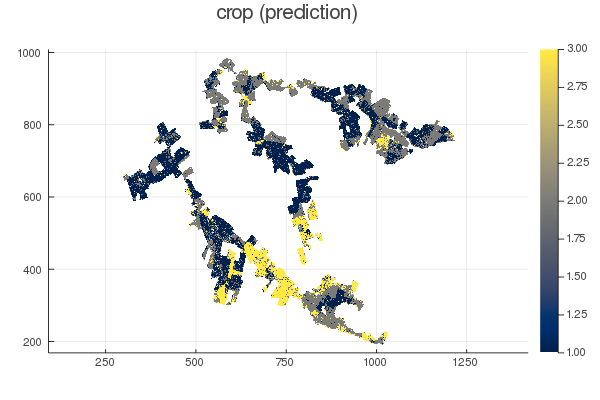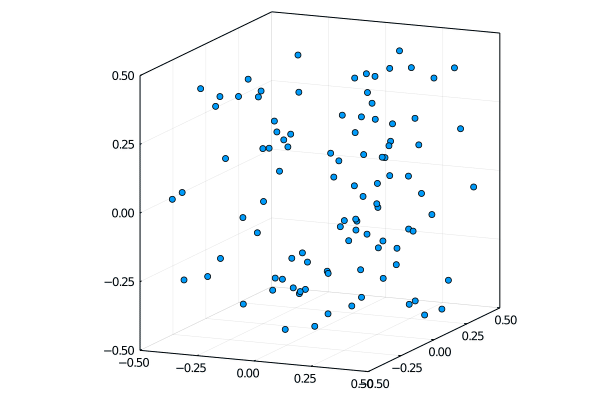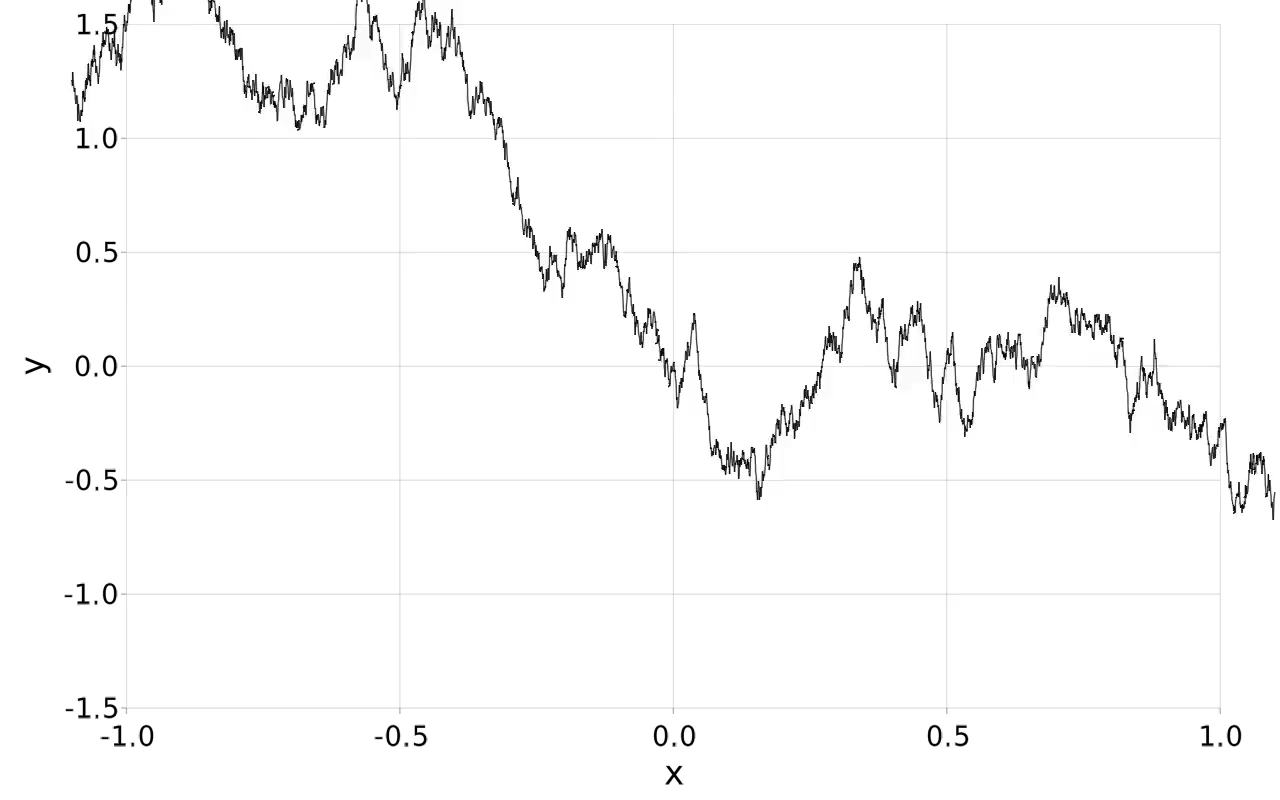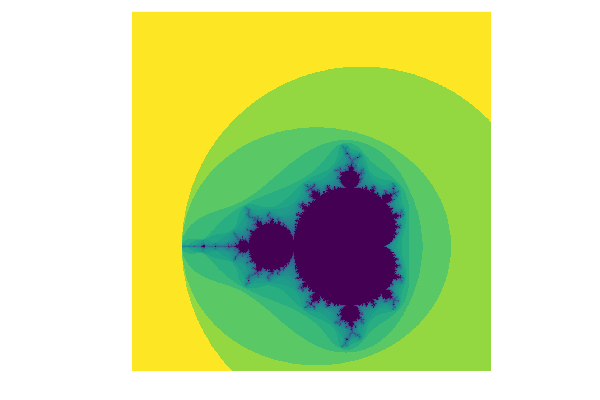# Seven Lines of Julia (examples sought)

Pretty much any of the examples in https://github.com/JuliaApproximation/ApproxFun.jl . Many are less then 7 lines already.

9 Likes

You may find this interesting! Was benchmarking the latest on Arrow with its work on DataFrames and a personal project I am working on:

Also, this is just nice to show the clarity of Julia and its ease of use:

4 Likes

You probably had the global variable `m` already defined before `using Unitful.DefaultSymbols`.

1 Like

I’m loving all the examples being presented here. I would like to encourage folks to submit as many different kinds of examples as possible, and I’ll work on collecting them and getting them onto julialang.org and generally on to social media with something like a #WhyJulia or #WorldOfJulia tag. I think @jiahao used to maintain such a thing a while ago for presentations and such.

It would greatly help to have a 3-5 line description of what it is doing, and why it is interesting, to go along with the code snippet.

-viral

26 Likes

The examples are nice and interesting, but IMO <10 LOC is not where Julia really shines. When not relying on packages, this domain is mostly about surface syntax tricks baked into the language.

The amazing powers of the language start to show around 100 LOC, and come into play fully from 1-5 kLOC, replicating functionality and speed of much larger libraries in other languages.

12 Likes

Agree with Tamas, but it is also nice to have a couple of very short examples. Here a Gibbs sampler sampling the posterior parameters of normal observations with mean distributed a priori as N(μ0, σ0) and precision 1/σ^2 a priori as Gamma(α, β):

``````using Distributions, Statistics
function mc(x, iterations, μ0 = 0., τ0 = 1e-7, α = 0.0001, β = 0.0001)
sumx, n = sum(x), length(x)
μ, τ = sumx/n, 1/var(x)
samples = [(μ, τ)]
for i in 1:iterations
μ = rand(Normal((τ0*μ0 + τ*sumx)/(τ0 + n*τ), 1/sqrt(τ0 + n*τ)))
τ = rand(Gamma(α + n/2, 1/(β + 0.5*sum((xᵢ-μ)^2 for xᵢ in x))))
push!(samples, (μ, τ))
end
samples
end
``````

Usage

``````samples = mc(rand(Normal(0.2, 1.7^(-0.5)), 1_000), 10_000)
μs, τs = first.(samples), last.(samples)
println("mean μ = ", mean(μs), " ± ", std(μs))
println("precision τ = ", mean(τs), " ± ", std(τs))
``````

Output:

`mean μ = 0.2076960556449643 ± 0.023693641548604788`
`precision τ = 1.8301418451485263 ± 0.08225999887566306`

9 Likes

Couldn’t this be written without the need for a loop and `push!`? I think this would get you to seven lines while also being to my eye quite readable:

``````using Distributions, Statistics

function mc(x, iterations, μ₀ = 0., τ₀ = 1e-7, α = 0.0001, β = 0.0001)
∑x, n = sum(x), length(x)
μ, τ = ∑x/n, 1/var(x)
μₛ = rand(Normal((τ₀*μ₀ + τ*∑x)/(τ₀ + n*τ), 1/√(τ₀ + n*τ)), iterations)
τₛ = rand(Gamma(α + n/2, 1/(β + 0.5*sum((xᵢ-μ)^2 for xᵢ in x))), iterations)
samples = [(μ, τ); collect(zip(μₛ, τₛ))]
end
``````
3 Likes

There is interdependence - the next value of `μ` depends on `τ` and vice versa. This is typical for a Gibbs sampler that you cannot parallelise it easily and really need a loop here (and this is exactly what brought me to try Julia in the first place)

8 Likes

Ah yes, I somehow thought the `μ` and `τ` would always come from the outer scope, and the results only differed on the fifth decimal or so when I checked so it seemed equivalent!

1 Like

More than 7 lines of code, but still a quite compact pipeline for geospatial machine learning:

``````using GeoStats, MLJ, CSV, Plots

df.crop = categorical(df.crop)

Ω = georef(df, (:x,:y))

Ωs, Ωt = split(Ω, 0.2, (1.0,-1.0))

𝒫 = LearningProblem(Ωs, Ωt, 𝒯)
𝔏 = PointwiseLearn(model)

Ω̂t = solve(𝒫, 𝔏)

plot(Ω̂t, ms=0.2, c=:cividis, title="crop (prediction)")
``````Commented example is here: https://juliaearth.github.io/GeoStats.jl/stable/workflow/#Defining-problems

16 Likes

Nice observation that the “wrong” solution is so good… Bernstein von Mises theorem strikes again.

4 Likes

Love everyone’s contributions… Hate to say it but I hard a hard time finding something around 7 LOC to submit :D. But, I did think of something I did a while back.

``````mutable struct βLASSO
η::Float32
λ::Float32
β::Float32
end

βLASSO(η = Float32(0.01), λ = Float32(0.009), β = Float32(50.0)) = βLASSO(η, λ, β)

function apply!(o::βLASSO, x, Δ)
Δ = o.η .* ( Δ .+ ( o.λ .* sign.( Δ ) ) )
Δ = Δ .* Float32.( abs.( x.data ) .> ( opt.β * opt.λ ) )
return Δ
end
``````

(full sloppy gist here: blasso gif.jl · GitHub)

Summary:
I was reading the following paper: https://arxiv.org/pdf/2007.13657.pdf around when it was first printed. The paper describes a projected gradient descent method for a penalized(β) LASSO regularization. In < 30min I was able to write my own optimizer, implement the majority of the paper and play with the loss function using Flux.jl. Below is a synthetic example of their loss function properly selecting a single variable of interest while minimizing the contributions of the others.Why Julia:
The tools in the ecosystem and the language facilitate quickly implementing cutting edge stuff. Plus, unicode support for readability!

7 Likes

@juliohm, the nice plot took very long time to display in my laptop (2nd time plotting it was also very slow). Any recommendation on this?

It may be an issue with Plots.jl @rafael.guerra, I had to lock the version to some old version in the documentation for example because plots became too slow. Are you also using the GR backend? We can try to address this in Zulip or in a private message.

Like with Julia, experts say that Shakespearean language can be tricky but that acting it out makes it fun and understandable. On the same vein, the following MIT list of Shakespearean insults might be a good way to learn both languages:

``````using CSV, DataFrames, HTTP
f = CSV.File(HTTP.get("http://web.mit.edu/dryfoo/Funny-pages/shakespeare-insult-kit.html").body);
sk = DataFrame(f[17:66])  # TODO: findfirst "artless" word to set range
r = size(sk,1)
sk = DataFrame([split(sk[i,1]) for i in 1:r])  # output = 3 rows x 50 columns
Shakespeare() = println("Thou "*sk[1,rand(1:r)]*" "*sk[2,rand(1:r)]*" "*sk[3,rand(1:r)])
``````

Then call Shakespeare:

``````julia> Shakespeare()
julia> Shakespeare()
Thou villainous motley-minded giglet
``````
17 Likes

With 15 lines I could write a code to perform a particle simulation with periodic boundary conditions, a langevin thermostat, and a quadratic potential between the particles, and produce an animation:

``````using Plots ; ENV["GKSwstype"]="nul"
const N, τ, Δt, λ, T, k = 100, 1000, 0.01, 1e-3, 0.26, 1e-6
const x, v, f = -0.5 .+ rand(3,N), -0.01 .+ 0.02*randn(3,N), zeros(3,N)
wrap(x,y) = (x-y) > 0.5 ? (x-y)-1 : ( (x-y) < -0.5 ? (x-y)+1 : (x-y) )
anim = @animate for t in 1:τ
f .= 0
for i in 1:N-1, j in i+1:N
f[:,i] .+= wrap.(x[:,i],x[:,j]) .- λ .* v[:,i]
f[:,j] .+= wrap.(x[:,j],x[:,i]) .- λ .* v[:,j]
end
x .= wrap.(x .+ v*Δt .+ (f/2)*Δt^2,zeros(3))
v .= v .+ f*Δt .+ sqrt.(2*λ*k*T*Δt)*randn()
scatter(x[1,:],x[2,:],x[3,:],label="",lims=(-0.5,0.5),aspect_ratio=1,framestyle=:box)
end
gif(anim,"anim.gif",fps=10)
``````As Tamas mentioned, if we go to a few more lines, this could include an actual Lennard-Jones potential and be parallelized. And that without using any package besides Plots.

55 Likes

I love watching particles dance! Awesome Leandro!

1 Like

Infinizoom! (Background: Simulates a Brownian motion and uses the Brownian self-similarity Wiener process - Wikipedia combined with simple data-augmentation/Brownian bridge sampling to zoom in infinitely.)

``````using Makie
dt = 0.0005; ϵ = 0.1; t = collect(-1.0:dt:1.0); n = length(t)
x = sqrt(dt)*cumsum(randn(n)); x .-= x[end÷2]
T, X = Node(t), Node(x)
p = lines(T, X); display(p)
xlims!(-1.0, 1.0); ylims!(-1.5,1.5)
while true
t .*= (1 + ϵ)
x .*= sqrt(1 + ϵ)
if t[end] > 2.0
t .= collect(-1.0:dt:1.0)
x[1:2:end] = x[n÷4+1:3n÷4+1]
x[2:2:end] = (x[1:2:end-2]+x[3:2:end])/2 + sqrt(dt)/2*randn(n÷2)
x[end÷2+1] = 0
end
T[] = t; X[] = x; sleep(0.02)
end
``````22 Likes

@FedericoStra, found interesting to perform a zoom-in loop and recompute your fractal and to use animated gif technique shown by @lmiq to create:``````xc, yc = -0.55, 0.61;
x0, x1 = xc - 2, xc + 2; y0, y1 = yc - 2, yc + 2;
anim = @animate for t in 1:50
x, y = range(x0, x1; length=1000), range(y0, y1; length=1000)
heatmap(x, y, -log.(mandelbrot.(x' .+ y .* im));aspect_ratio=1,border=:none,legend=:none);
x0, x1 = (15x0 + x1)/16, (15x1 + x0)/16;  y0, y1 = (15y0 + y1)/16, (15y1 + y0)/16
end
gif(anim,"mandelbrot_zooms.gif",fps=10)
``````
15 Likes

for reference, what is the extended code that gives your example (a) parallelized (b) with the actual L-J potential (c) both

1 Like